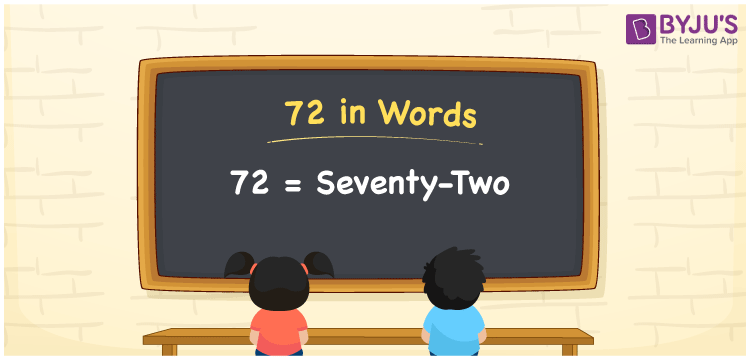# 72 in Words

The number 72 in words is Seventy-two. The number 72 can be converted to words easily using the place value of a given number. As a result, you’ll need to use a place value table to make the number names. For instance, if you bought a book for Rs. 72, you may write “I bought a book for Rs. Seventy-two”. Here, you will learn how to spell 72 in English and how to express the number in words in detail.

 72 in Words: Seventy-two. Seventy-two in Numerical Form: 72.

## 72 in English Words## How to Write 72 in Words?

To convert numbers to words, use a place value chart. Below is a place value chart for the number 72.

 Tens Ones 7 2

Below is a representation of the number 72 in its expanded form.

= 7 × Ten + 2 × One

= 7 × 10 + 2 × 1

= 70 + 2

= 72

= Seventy-two

Hence, 72 in words is Seventy-two.

The natural number 72 appears after 71 but before 73.

72 in words – Seventy-two

Is 72 an odd number? – No

Is 72 an even number? – Yes

Is 72 a perfect square number? – No

Is 72 a perfect cube number? – No

Is 72 a prime number? – No

Is 72 a composite number? – Yes

## Frequently Asked Questions on 72 in Words

Q1

### How to write 72 in words?

72 in words is seventy-two.

Q2

### Simplify 80 – 8, and express in words.

Simplifying 80 – 8, we get 72. Hence, 72 in words is seventy-two.

Q3

### Convert seventy-two into numbers.

Seventy-two in numbers is 72